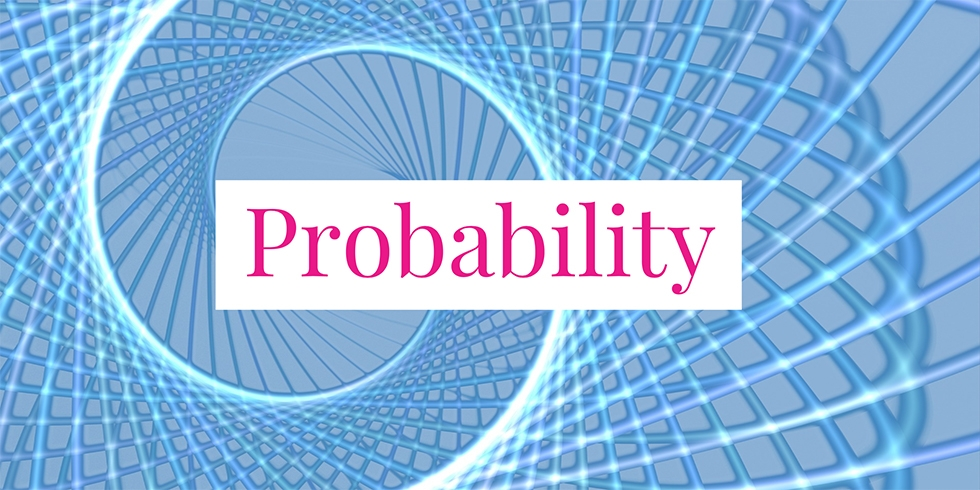# Probability of Getting Two Red Balls From the Chosen Box## Problem 751

There are two boxes containing red and blue balls. Let us call the boxes Box A and Box B. Each box contains the same number of red and blue balls. More specifically, Box A has 5 red balls and 5 blue balls. Box B has 20 red balls and 20 blue balls. You choose one box. Then draw two balls randomly from the chosen box without replacement, that is, you will not return the first ball into the box before picking up the second ball.

If you draw two balls with the same color, then you win. Otherwise, you lose. To maximize the chance of winning, which box should you pick?Add to solve later

Contents

## Solution.

Let us consider the probability that you draw two red balls from the box you choose.
Since both boxes contain the same number of red and blue balls, the probability that your first ball is red is $1/2$ no matter which box you choose. More rigorously, if you choose Box A, then the probability that the first ball is red is $5/10 = 1/2$. Also, if you choose Box B, then the probability is $20/40=1/2$.

Thus, the difference (if any) comes from the probability of the second ball. Suppose that you choose Box A. Assume that your first ball was red. Then Box A currently contains 4 red balls and 5 blue ball. Hence the probability that the second ball is red is $4/9$.
Similarly, if you choose Box B and the first ball is red, then the probability that the second ball is red is $19/39$ since there are 19 red balls and 20 blue balls in Box B.

So, which probability is bigger? As we have
$4/9 = 0.444\dots \text{ and } 19/39 = 0.487\cdots$ The probability for Box B is bigger. Thus, the probability of getting two red ball is higher when you choose Box B. The same argument applies for two blue balls. Therefore, to maximize the chance of winning, you should select Box B.

## Remark.

Let us give an intuitive solution. This approach is not mathematically rigorous but it might help you reach the conclusion quicker.
First, you may think the actual numbers $5$ and $20$ are not so importance. What is importance is which number is bigger. So, instead, we consider extremely $2$ and $2,000,000,000$ (any gigantic number is fine). So Box A contains only 2 red balls and 2 blue balls. On the other hand Box B contains $2,000,000,000$ balls for each color.

As before the probability of getting the first red ball is the same regardless of your choice of a box. For the second ball, however, there is only one red ball remained in Box A, which is a half of the number of blue balls in Box A. On the other hand, Box B still contains 1,999,999,999 red balls and 2,000,000,000 blue balls. The ratio of two colors in Box B is almost 1. Thus, you have a better chance of getting the second red ball if you choose Box B.Add to solve later

### More from my site

#### You may also like...

This site uses Akismet to reduce spam. Learn how your comment data is processed.

###### More in Probability##### Coupon Collecting Problem: Find the Expectation of Boxes to Collect All Toys

A box of some snacks includes one of five toys. The chances of getting any of the toys are equally...

Close